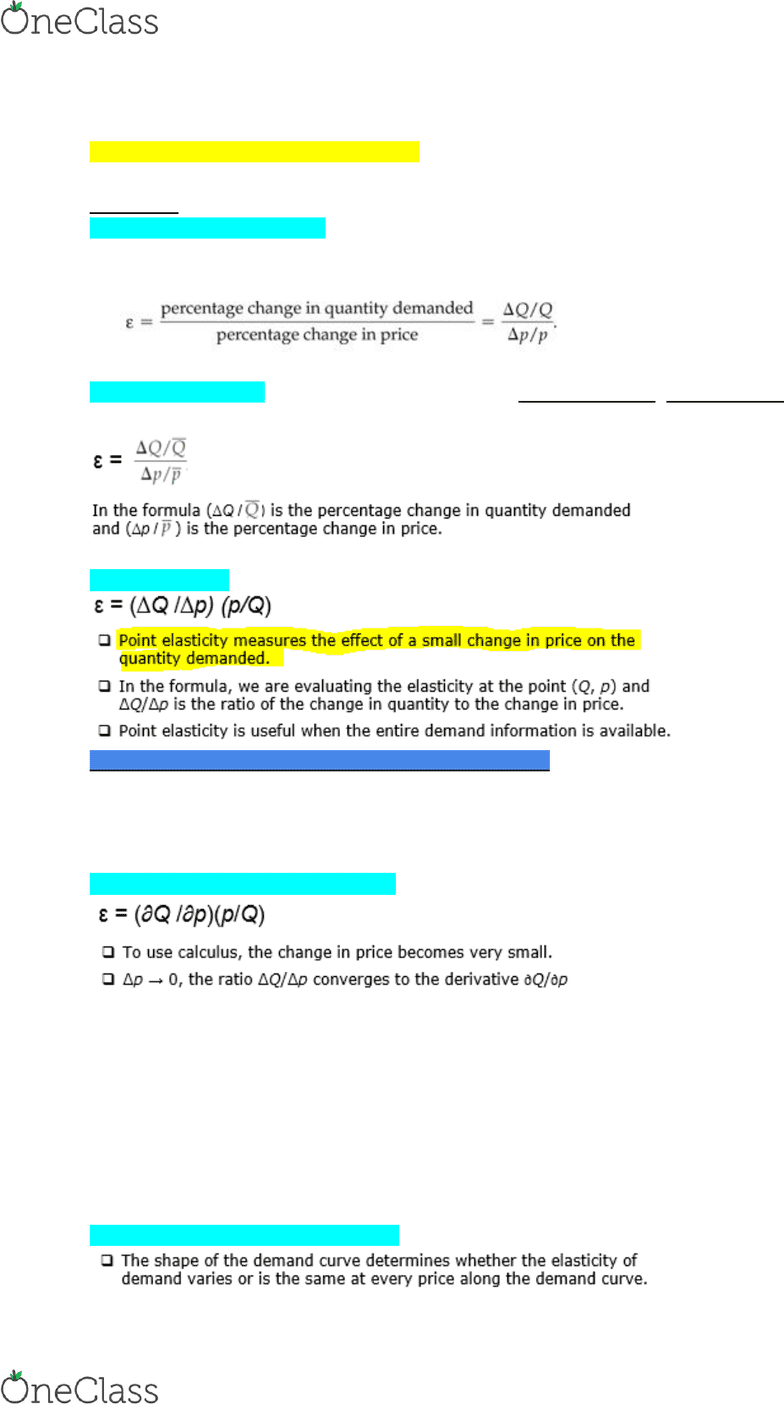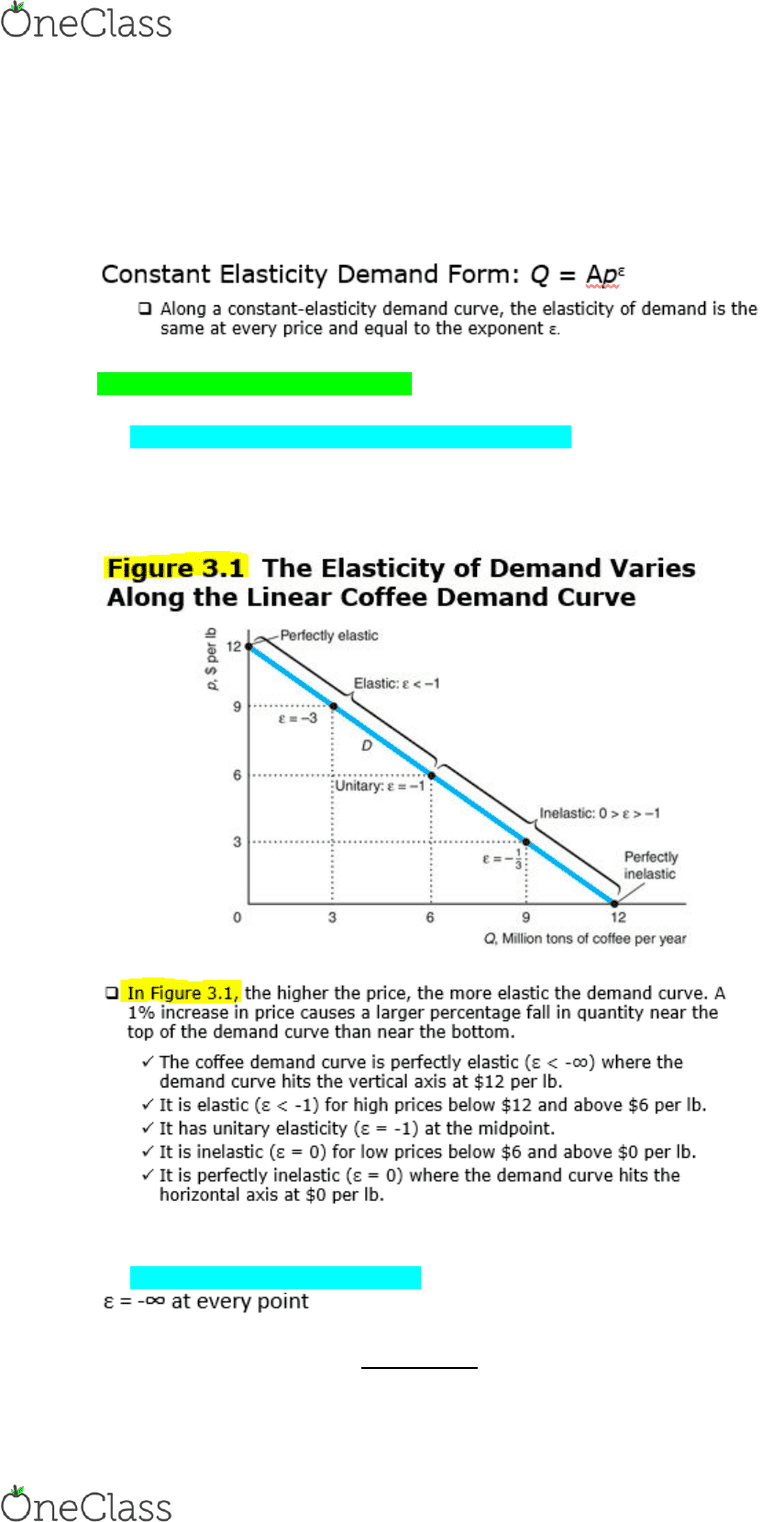Class Notes (1,100,000)
CA (650,000)
SFU (10,000)
BUS (1,000)
BUS 207 (20)
Lecture 2

# BUS 207 Lecture Notes - Lecture 2: Negative Number, Dependent And Independent Variables, Regression Analysis

Department
Course Code
BUS 207
Professor
Mark Moore
Lecture
2

This preview shows pages 1-2. to view the full 7 pages of the document.BUS 207: Chapter 3
Empirical Methods for Demand Analysis
_________________________________________________________________________
Elasticity
Price elasticity of demand: Is the percentage change in quantity demanded (Q) divided by
the percentage change in price (P).
Arc Price Elasticity: It is an elasticity that uses the average quantity, average price, as the
denominators for percentage calculations.
Point Elasticity:
***We can be given an inverse function of the original***
P = 10 - 1/2q ...... We cannot take the derivative of this...
Q = 20 - 2p ...... We rearranged the question above to isolate Q, quantity ... take derivative
SQ/SP = -2
Point Elasticity (WITH CALCULUS)
Elasticity Along the Demand Curve
find more resources at oneclass.com
find more resources at oneclass.com

Only pages 1-2 are available for preview. Some parts have been intentionally blurred.3 types of linear demand curves:
1. Downward-sloping
2. Horizontal
3. Vertical
_________________________________________________________________________
3 types of linear demand curves:
1. Downward-Sloping Linear Demand Curves
Elasticity varies along the demand curve.
Elasticity of demand is a more negative number the higher the price and hence the
smaller the quantity.
2. Horizontal Demand Curves
If the price increases, even slightly, demand falls to zero.
find more resources at oneclass.com
find more resources at oneclass.com